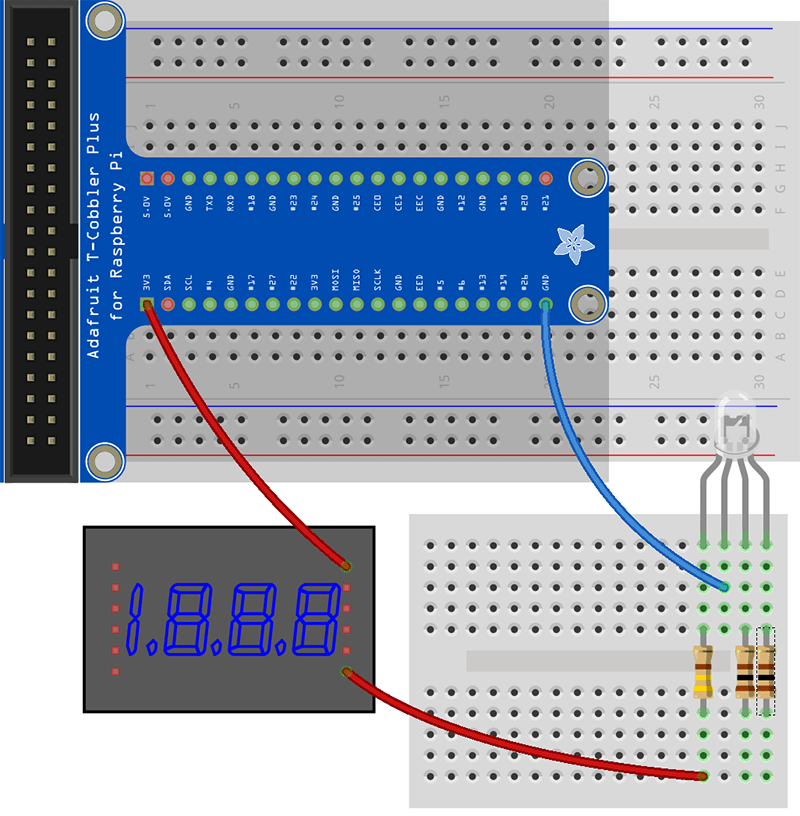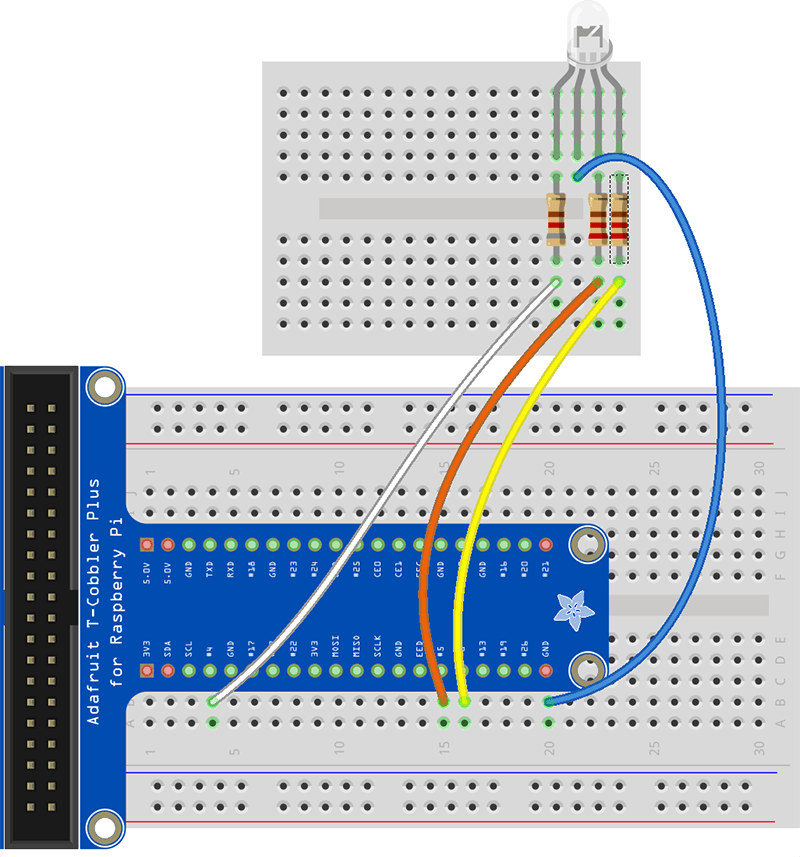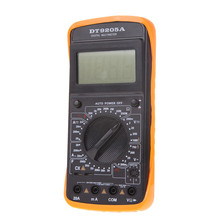# Working with LED’s – And Wiring 4 pin RGB led to Raspberry PI

## In this example we are going learn some basics on how to calculate the resistor for LED, and we will take a look at how to wire up a 4 pin RGB LED.

Disclaimer:
We do not take any responsibility for possible errors in the guide or errors that you might do wiring it up. Incorrect wiring can result in damaged sensor or damaged Raspberry PI.

##### Pieces we use are:
• Raspberry PI 2 or Raspberry PI 3
• Cobbler and Cobbler cable
• Bread board (Big one and mini breadboard but the big one would be enough)
• 4 pin RGB LED.
• Some resistors (types depend on spec of your RGB led)
• We are going to be using digital multi-meter to verify the spec of the LED and to verify our calculations. But if you know the specs of your LED for sure then you will not be needing this. (Digital multimeter is really one of the first things you should have if you are going to be playing around with electronics. Their cheap you can get good one for around \$15 to \$20. Mine was bought in Kiel in Germany right at the time when the endless lines of Trabants started to flow from East Germany to the West, so they really can last you for long time)
• Wiring PI Xojo module from Paul Lefebvre (Note we will be making modifications for the interrupts example)

If you are not familiar with breadboards, how to place a cobbler on the breadboard or need to know how to read the color codings on the resistors then click here bellow

First thing is to understand the basics of LED.

You need to know the spec of your LED, important numbers are Vf and how much maximum current they can handle.

The Vf is the forward voltage of the LED.  This is important when you put it into the Ohms equations.

The GPIO pin on Raspberry PI will have around 3.3 V and the absolute maximum you may take from it is 16 mA, peek usage, meaning the pins were designed for about 3 mA if all are in use.

So to calculate the resistor needed then you need to know Vr which is the Voltage on the circuit minus forward voltage of the LED (Vf). Then we can apply the Ohms law:

I = V / R

which can be re-written as:

R = V / I

Where V is Voltage of the circuit – Vf of the LED and I is desired current.

We are in this example going to aim for 5 mA.

##### Wiring of the RGB 4 pin led:

If we first look at normal LED in the picture bellow then you can see that you have two ways to identify the + and – or anode vs cathode. Its the length of the pins where the longer wire is the anode and the shorter is the cathode. If wires have been trimmed then you can usually also see it on the LED it self the flat side on the edge is the cathode or – as is shown on the picture to right here.For RGB led then things get a bit more complicated. RGB leds usually share common cathode (black which would be the longest wire or you can see it inside the glass by the shape there if the wire has been shortened), and then three anodes, red on the left then greed right of the cathode and then blue on far right.(To complicate it a bit then I have seen RGB led where the anode was shared and there were three cathodes)

I thought the spec of the led that I used was around 2 Vf for Red with max 50mA current. and 3 Vf for green and blue with max of 30 mA
(Check specs on your LED if you have data before calculating) (See bellow, where I was incorrect on the spec)

We apply this spec to the equations shown above where we decided on taking 3 mA from the pin.

R(red) = (3.3 – 2.0) / 0.005 = 433 Ω => 440 Ω
R(green) = 3.3-3.0) / 0.003 =100Ω
R(blue) = (3.3-3.0) / 0.003 = 100 Ω

Unless you got 5 band resistor that is 433 Ω then use normal 4 band one that is   440 Ω. We went with 5 band 433 Ω

Now I was not  sure of the spec I had for the RGB LED as I had just guessed it from fairly normal values but I didnt know, so I proceeded with caution, instead of going for GPIO’s right away then I decided to first just connect those resistors and the LED to the 3,3 V output on the Rapsberry PI along with a Digital Multimeter in between to measure the current I would draw with this setup.So I just just 3,3 V output and GND, on the LED with a Digital multimeter to measure the current draw. The connection was done as shown in the drawing above, connecting to one resistor at a time to check their current draw.

I had the LED on mini bread board because of this middle step it was easier to deal with it.

Note that the 3,3 V output has maximum draw of 50 mA so you cannot do tests like this on just anything.

It was soon obvious that the LED I had had totally different spec than expected, I ended with 820 Ω for the red and 220Ω for the others, then I had values I was confortable putting on the GPIO.

After the calibration with the multi-meter then we had the following resistors:

R(red) =  820Ω
R(green) =220Ω
R(blue) = 220 Ω

(If only having calculating values then its not bad idea to go with a little higher value resistors than you calculated to protect the pins just in case)

Once I was satisfied with the values read from the multi-meter and convinced that it would not draw to much from the Raspberry GPIO pin then I proceeded in wiring the GPIO pins to the LED.

I selected GPIO 4, for Red anode, GPIO  5 for Green anode, GPIO 6 for Blue anode.# The Xojo code for this one is simple

We had 3 checkboxes on a window, with the texts, Red, Green, Blue.

The open event of the window:

```Sub Open()
GPIO.SetupGPIO
GPIO.PinMode(4,GPIO.OUTPUT)
GPIO.PinMode(5,GPIO.OUTPUT)
GPIO.PinMode(6,GPIO.OUTPUT)

GPIO.DigitalWrite(4,GPIO.OFF)
GPIO.DigitalWrite(5,GPIO.OFF)
GPIO.DigitalWrite(6,GPIO.OFF)
End Sub```

The check box events:

Red checkbox

```Sub Action()
if me.Value then
GPIO.DigitalWrite(4,GPIO.ON)
else
GPIO.DigitalWrite(4,GPIO.OFF)
end if
End Sub```

Green checkbox

```Sub Action()
if me.Value then
GPIO.DigitalWrite(5,GPIO.ON)
else
GPIO.DigitalWrite(5,GPIO.OFF)
end if
End Sub```

Blue checkbox

```Sub Action()
if me.Value then
GPIO.DigitalWrite(6,GPIO.ON)
else
GPIO.DigitalWrite(6,GPIO.OFF)
end if
End Sub```

Thats it for now. I will probably make a followup on this one adding transistors maybe to see how more current can be given to the LED without hurting the GPIO pins.

```AliExpress.com Product - Brand digital multimeter multimetro tester AC DC LCD Display Professional Electric Handheld Testers multimetr free shipping ```

```AliExpress.com Product - Carbon film resistor assorted kit 0.33 ohm - 4.7M ohm 1/4w 5% tolerance commonly used 122 values 20x 122= 2440pcs ```